NEET  >  Test: Packing Efficiency

# Test: Packing Efficiency

Test Description

## 10 Questions MCQ Test Chemistry Class 12 | Test: Packing Efficiency

Test: Packing Efficiency for NEET 2022 is part of Chemistry Class 12 preparation. The Test: Packing Efficiency questions and answers have been prepared according to the NEET exam syllabus.The Test: Packing Efficiency MCQs are made for NEET 2022 Exam. Find important definitions, questions, notes, meanings, examples, exercises, MCQs and online tests for Test: Packing Efficiency below.
Solutions of Test: Packing Efficiency questions in English are available as part of our Chemistry Class 12 for NEET & Test: Packing Efficiency solutions in Hindi for Chemistry Class 12 course. Download more important topics, notes, lectures and mock test series for NEET Exam by signing up for free. Attempt Test: Packing Efficiency | 10 questions in 15 minutes | Mock test for NEET preparation | Free important questions MCQ to study Chemistry Class 12 for NEET Exam | Download free PDF with solutions
 1 Crore+ students have signed up on EduRev. Have you?
Test: Packing Efficiency - Question 1

### For which type of cubic lattice, a = 2r?

Detailed Solution for Test: Packing Efficiency - Question 1

In a simple cubic lattice, the side of the solid structure is equal to twice the radius of the atom.

Hence the correct answer is simple cubic.

Additional Information: A cubic lattice is a lattice whose points lie at positions (x,y,z) in the Cartesian three-space, where x, y, and z are integers.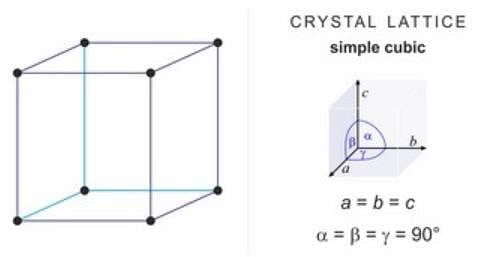There are three main types of cubic lattices:

1. the simple cubic structure (sc) (also known as primitive cubic)

2. the body-centred cubic structure (bcc)

3. the face-centred cubic structure(fcc)

Test: Packing Efficiency - Question 2

### Packing efficiency of body centred cubic unit cell is:

Detailed Solution for Test: Packing Efficiency - Question 2

The packing efficiency of a body-centred cubic unit cell is 68%.

Explanation:

The packing efficiency is the fraction of the crystal (or unit cell) actually occupied by the atoms. It is usually represented by a percentage or volume fraction.

Mathematically Packing Efficiency is:

Number of atoms × volume occupied by 1 share × 100/Total volume of unit cell × 100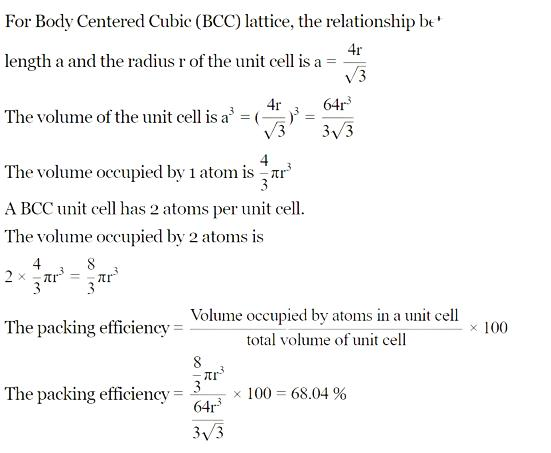Test: Packing Efficiency - Question 3

### Packing efficiency in a unit cell is never 100% because constituent particles are assumed to be:

Detailed Solution for Test: Packing Efficiency - Question 3

Packing efficiency can never be 100% because in packing calculations all constituent particles filling up the cubical unit cell are assumed to be spheres.

Test: Packing Efficiency - Question 4

If r is radius of void and R is radius of the sphere, then for an atom to ocuppy an octahedral void which relation holds true?

Detailed Solution for Test: Packing Efficiency - Question 4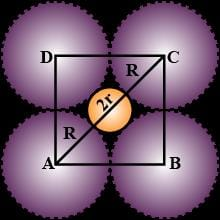Let the radius of the octahedral void be r and radius of the atoms in close-packing be R and the edge length be a.

In the right angle triangle ABC,

AB = BC = a

For the diagonal AC,

Using Pythagoras Theorem we get;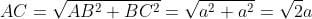Also,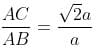And, AB = 2R

AC = R + 2r + R = 2R + 2r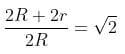r = 0.414R

Hence, the relation is r = 0.414R.

Test: Packing Efficiency - Question 5

Which type of void is represented by the following diagram?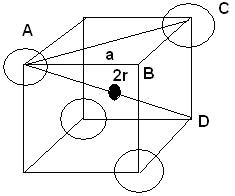Detailed Solution for Test: Packing Efficiency - Question 5

A tetrahedral void is formed when one sphere or particle is placed in the depression formed by three particles.

Definition of Tetrahedral Void: The vacant space or void among the four constituent particles having a tetrahedral arrangement in the crystal lattice is called tetrahedral void.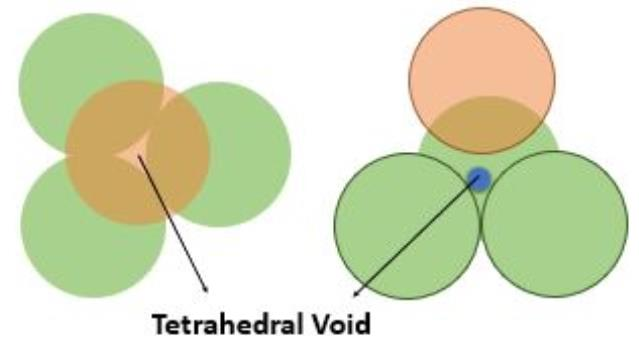Test: Packing Efficiency - Question 6

HCP structure is present in:

Detailed Solution for Test: Packing Efficiency - Question 6
• Magnesium has a hexagonal close-packed (HCP) crystal structure.
• Bonding is non-directional metallic bonding.
• Magnesium is metallic solid. The units occupying lattice sites are Mg ions and these ions are surrounded by mobile or delocalized electrons.
• The arrangement of ions in one plane the arrangement of ions is a hexagonal array or closed packed layer. Thus each metal ion is touching six adjacent ions in one plane.
• Metals that crystallize in an HCP structure include Cd, Co, Li, Mg, Na, and Zn, and metals that crystallize in a CCP structure include Ag, Al, Ca, Cu, Ni, Pb, and Pt.

Test: Packing Efficiency - Question 7

If r is the radius of the void and R is the radius of the sphere, what is AC in the following diagram?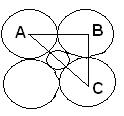Detailed Solution for Test: Packing Efficiency - Question 7

AC = Diameter of void + (2× Radius of sphere)

AC = 2r + 2R = 2(R+r)

Therefore the correct answer is B.

Test: Packing Efficiency - Question 8

Which of the following shows maximum packing efficiency?

Detailed Solution for Test: Packing Efficiency - Question 8

Packing efficiency is defined as the percentage of space occupied by constituent particles packed inside the lattice.

Packing efficiency = Number of atoms × volume occupied by one share × 100/total volume of the unit cell

• Packing efficiency in fcc and hcp structures is 74%

• The packing efficiency of the body-centred unit cell is 68%.

• The packing efficiency of the simple unit cell is 52.4%

Since HCP shows the maximum packing efficiency, the correct answer is A.

Test: Packing Efficiency - Question 9

The number of atoms in each unit cell in ccp structure is:

Detailed Solution for Test: Packing Efficiency - Question 9

In ccp structure or fcc structure, the number of atoms per unit cell is calculated as follows

Thus, in a face-centred cubic unit cell (fcc) or cubic close packing (ccp), we have

8 corners × 1/8 per corner atom = 8 × 1/8 = 1 atom

6 face-centred atoms × 1/2 atom per unit cell = 3 atoms,

Therefore, the total number of atoms in a unit cell = 4 atoms.

Test: Packing Efficiency - Question 10

The number of atoms per unit cell in a body centred cubic arrangement is:

Detailed Solution for Test: Packing Efficiency - Question 10

In a Body-centred cubic arrangement, there are 8 corners and 1 corner shares 1/8th the volume of the entire cell, so:  8×1/8

8×18 = 1 atom

There is also 1 atom at the centre of the body of the cube. This can’t be shared. So, it will stay as one atom whole: 1 × 1 = 1 atom

Total atoms = 1 + 1 = 2 atoms

So, from this, we can say that the number of atoms in the unit cell of the body-centred cubic crystal is 2.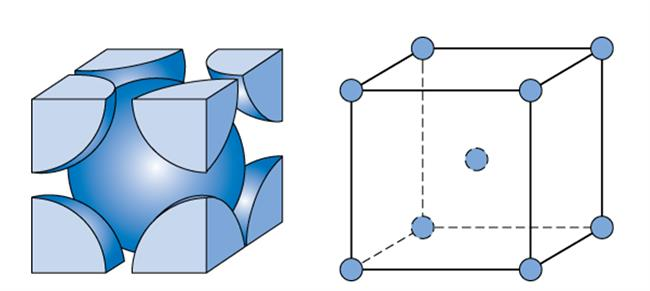## Chemistry Class 12

157 videos|362 docs|261 tests
 Use Code STAYHOME200 and get INR 200 additional OFF Use Coupon Code
Information about Test: Packing Efficiency Page
In this test you can find the Exam questions for Test: Packing Efficiency solved & explained in the simplest way possible. Besides giving Questions and answers for Test: Packing Efficiency, EduRev gives you an ample number of Online tests for practice

## Chemistry Class 12

157 videos|362 docs|261 tests

### How to Prepare for NEET

Read our guide to prepare for NEET which is created by Toppers & the best Teachers